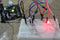# Light

Whilst getting input from a potentiometer can be useful for human controlled experiments, what do we use when we want an environmentally controlled experiment? We use exactly the same principles but instead of a potentiometer (twist based resistance) we use a photo resistor (light based resistance). The Arduino cannot directly sense resistance (it senses voltage) so we set up a voltage divider. The exact voltage at the sensing pin is calculable, but for our purposes (just sensing relative light) we can experiment with the values and see what works for us. A low value will occur when the sensor is well lit while a high value will occur when it is in darkness.

### Hardware Used:

• 1 – Light Dependent Resistor

• 1 – LED
•

• 1 – 10k Resistor
• 1 – 100 ohm Resistor
•

Arduino IDE

### Set up the Hardware:### Code:

```/*
* A simple programme that will change the intensity of
* an LED based  * on the amount of light incident on
* the photo resistor.
*
*/

//PhotoResistor Pin
int lightPin = 0;
//LED Pin
int ledPin = 9;   //the pin the LED is connected to
//we are controlling brightness so
//we use one of the PWM (pulse width
// modulation pins)
void setup()
{
pinMode(ledPin, OUTPUT); //sets the led pin to output
}
/*
* loop() - this function will start after setup
* finishes and then repeat
*/
void loop()
{
lightLevel = map(lightLevel, 0, 900, 0, 255);

lightLevel = constrain(lightLevel, 0, 255);
analogWrite(ledPin, lightLevel);```

### Code Breakdown:

`int lightPin = 0;`

the analog Pin for the photoresistor. this is simply a raw sensor value (relative light)

`int ledPin = 9;`

the pin the LED is connected to. we are controlling brightness so we use one of the PWM (pulse width modulation) pins

`pinMode(ledPin, OUTPUT);`

sets the led pin to output

`int lightLevel = analogRead(lightPin);`

Read the lightlevel. analogRead() Reads the value from the specified analog pin. This means that it will map input voltages between 0 and 5 volts into integer values between 0 and 1023.

`lightLevel = map(lightLevel, 0, 900, 0, 255);`

map() = Re-maps a number from one range to another. That is, a value of fromLow would get mapped to toLow, a value of fromHigh to toHigh, values in-between to values in-between, etc.

map(value, fromLow, fromHigh, toLow, toHigh)

## Try MOREEE!!!!

### Reverse the response:

Perhaps you would like the opposite response. Don’t worry we can easily reverse the response just change:

`analogWrite(ledPin, lightLevel); ----> analogWrite(ledPin, 255 - lightLevel);`

Upload and watch the response change.

### Night light:

Rather than controlling the brightness of the LED in response to light, let’s instead turn it on or off based on a threshold value. Change the loop() code with.

```void loop() {
int threshold = 300;
digitalWrite(ledPin, HIGH);
} else {
digitalWrite(ledPin, LOW);
}
}```

The post Arduino Starter’s Guide (4/7): Light Dependent Resistor and LED appeared first on CreateLabz.